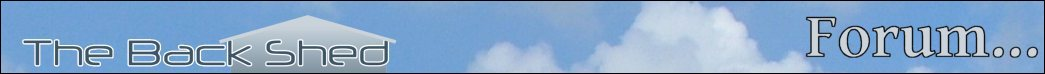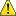Notice. New forum software under development. It's going to miss a few functions and look a bit ugly for a while, but I'm working on it full time now as the old forum was too unstable. Couple days, all good. If you notice any issues, please contact me.

Forum Index : Microcontroller and PC projects : CMM2 demo programs

Page 1 of 4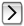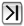Author Message
TassyJim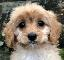Guru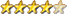Joined: 07/08/2011
Location: Australia
Posts: 5416
 Posted: 03:54am 21 May 2020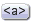Copy link to clipboard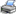Print this post

During the testing of the CMM2, I needed a few short programs designed to demonstrate some of the features.
This rotating cube program started life in a QuickBasic forum.

To change it to MMBasic the only changes needed were PSET changed to PIXEL and  BOX used to erase the frames.
I then used PAGE to remove the ficker and a timer so you can compare the different graphic MODEs

A couple of tweaks to the formula shaved about 10mS of each frame.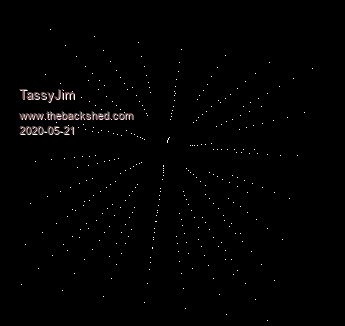' cube rotator
' From a 19 liner by Entropy, shrinked by Antoni Gual
' for Rel's 9 LINER contest at QBASICNEWS.COM  1/2003
' translated to MMBasic for the CMM2 by TassyJim May 2020

DIM INTEGER x,y,z
DIM FLOAT r,x1,y1
DIM FLOAT COSr,SINr,denom,xyz
DIM INTEGER cx = MM.HRES/2  'scale things for different display resolution
DIM INTEGER cy = MM.VRES/2
DIM INTEGER b = cx/2
DIM INTEGER s = cx*3/4
CLS

DO
TIMER = 0
PAGE WRITE 1
'cls
BOX b,cy-b,cx,cx,1,0,0 ' 1mS quicker than CLS
r = (r + .01745) + 6.283185 * (r >= 6.283185)
COSr = COS(r)
SINr = SIN(r)
FOR x = -30 TO 30 STEP 10
FOR y = -30 TO 30 STEP 10
FOR z = -30 TO 30 STEP 10
denom = x*SINr+(z*COSr-y*SINr)*COSr+100 ' saves about 4.5mS
xyz = x*COSr-(z*COSr-y*SINr)*SINr       ' this one saves 3mS
x1 =(xyz*COSr+(y*COSr+z*SINr)*SINr)/denom
y1 =((y*COSr+z*SINr)*COSr-xyz*SINr)/denom
PIXEL (s * x1 + cx), (s * y1 + cy), RGB(WHITE)
NEXT z
NEXT y
NEXT x
PAGE WRITE 0
BLIT b,cy-b, b,cy-b,cx,cx ,1
TEXT cx,cy+b,STR\$(TIMER)+"mS",cm
LOOP UNTIL INKEY\$ <>""

' formula before speed refinements
' x1 =((x*COS(r)-(z*COS(r)-y*SIN(r))*SIN(r))*COS(r)+(y*COS(r)+z*SIN(r))*SIN(r))/(x*SIN(r)+(z*COS(r)-y*SIN(r))*COS(r)+100)
' y1 =((y*COS(r)+z*SIN(r))*COS(r)-(x*COS(r)-(z*COS(r)-y*SIN(r))*SIN(r))*SIN(r))/(x*SIN(r)+(z*COS(r)-y*SIN(r))*COS(r)+100)

Jim
VK7JH
MMedit   MMBasic Help

TassyJimGuruJoined: 07/08/2011
Location: Australia
Posts: 5416
 Posted: 07:35am 21 May 2020Copy link to clipboardPrint this post

Sudoku
This program incorporates a method of solving the puzzle which I found in the QB64 samples.
The code was very condensed which makes understanding the process difficult (for me).
It also has lots of GOTOs which I have only partially removed.

It does succeed most of the time.

MMBasic has limits on the amount of recursion you can do so the more usually solving algorithms were not available.

I could have cheated by saving a copy of the solved puzzle before starting but that�s not fair.

At any time, the �H� key toggles hints on/off
�Enter� lets the CCM2 solve it for you.

600 lines so I ZIPed it.

sudoku.zip

Jim
VK7JH
MMedit   MMBasic Help

TassyJimGuruJoined: 07/08/2011
Location: Australia
Posts: 5416
 Posted: 07:47am 21 May 2020Copy link to clipboardPrint this post

I started writing my File Manager before the inbuilt FILES command was expanded and decided to keep going.
It has been useful to understand and test how MMBasic on the CMM2 handles the various file types.

I have put in a few tests to catch some of the files that MMBasic doesn't handle such as oversize images but there are still plenty of ways to cause it to crash.

'h' for help

FM.zip

Jim
VK7JH
MMedit   MMBasic Help

GrogsterJoined: 31/12/2012
Location: New Zealand
Posts: 8593
 Posted: 09:02am 21 May 2020Copy link to clipboardPrint this post

Cube demo is EXCELLENT, Jim!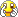Smoke makes things work. When the smoke gets out, it stops!

TassyJimGuruJoined: 07/08/2011
Location: Australia
Posts: 5416
 Posted: 11:33pm 21 May 2020Copy link to clipboardPrint this post

ColourBars is a short program to test all available graphics modes.
It can be tricky to set up a monitor to display all modes without trimming off pixels from one edge in some modes.
This program helps.
It also lets you find the optimum aspect ratio needed for the CIRCLE command. This will vary between monitors.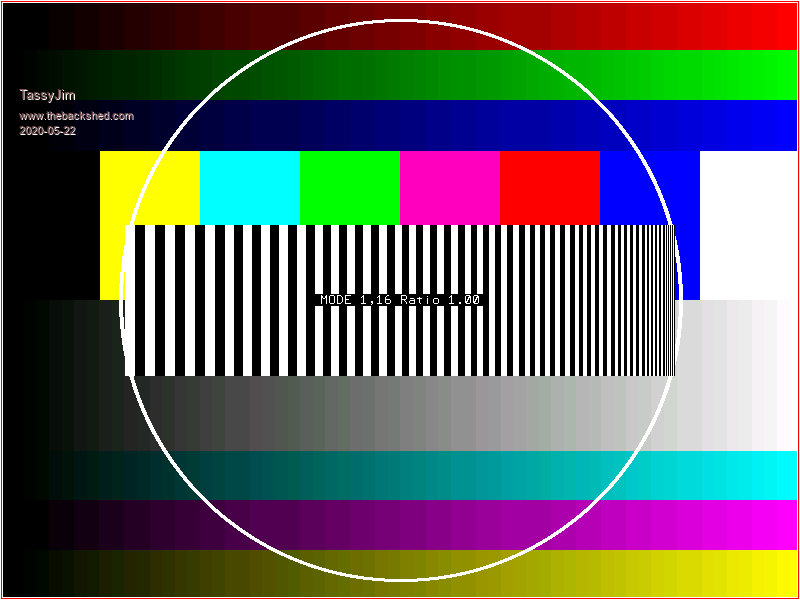colourBars.zip

Jim
VK7JH
MMedit   MMBasic Help

TassyJimGuruJoined: 07/08/2011
Location: Australia
Posts: 5416
 Posted: 05:59am 22 May 2020Copy link to clipboardPrint this post

This is one that serves no purpose at all.

To change it from QB64, I added the colours array and changed PSET to PIXEL and set the MODE

'sinecube QB64 2006 mennonite
'translated to MMBasic by TassyJim May2020

DIM blox(40, 40, 40) AS INTEGER
DIM col(15) ' GWBasic colours
col(0) = RGB(BLACK)
col(1) = RGB(BLUE)
col(2) = RGB(GREEN)
col(3) = RGB(CYAN)
col(4) = RGB(RED)
col(5) = RGB(MAGENTA)
col(6) = RGB(150, 75, 0)    ' brown
col(7) = RGB(192,192,192)   ' dull white
col(8) = RGB(127,127,127)   ' grey
col(9) = RGB(173, 216, 230) ' light blue
col(10) = RGB(173, 216, 230)' light green
col(11) = RGB(144, 238, 144)' light cyan
col(12) = RGB(255, 100, 100)' light red
col(13) = RGB(255, 120, 255)' light magenta
col(14) = RGB(YELLOW)       ' yellow
col(15) = RGB(WHITE)        ' bright white

MODE 2,16
CLS

B\$ = "00000000...llnnnnnnl..l8lnnnnnnl.l88lllllllll88l000000ll88l00000"
B\$ = B\$ + "0ll88l000000ll88l000000l.l8l000000l..ll000000l...llllllll"
l = 8
blox(2, 3, 32) = 1

FOR l = 8 * 32 TO 1 STEP -8
FOR y = 4 TO 4 * 32 STEP 4
FOR x = 8 * 32 TO 1 STEP -8
mm = SIN(x * y * l * 3.14): IF mm<0 THEN mm=-1 ELSE IF mm>0 THEN mm=1
IF blox(x / 8, y / 4, l / 8) = mm + 1 THEN
FOR by = 1 TO 11
FOR bx = 1 TO 11
IF RIGHT\$(LEFT\$(b\$,(by - 1) * 11 + bx),1) <> "." THEN
z = 11
c = ASC(RIGHT\$(LEFT\$(b\$,(by - 1) * 11 + bx),1)) MOD 16 + (y MOD 2)
PIXEL x + bx - 1 + y - 3, by - 1 + y + l + 4, col(c)
END IF

NEXT bx
NEXT by
END IF
IF INKEY\$ = CHR\$(27) THEN END
NEXT x
NEXT y
NEXT l

mode 1,8

Jim
VK7JH
MMedit   MMBasic Help

TassyJimGuruJoined: 07/08/2011
Location: Australia
Posts: 5416
 Posted: 06:57am 22 May 2020Copy link to clipboardPrint this post

I really need to get out more:

'Lissajous by Antoni Gual
' for Rel's 9 LINER contest at QBASICNEWS.COM  1/2003
' converted to MMBasic for the CMM2 by TassyJim May 2020

DIM col(15) ' GWBasic colours
col(0) = RGB(BLACK)
col(1) = RGB(BLUE)
col(2) = RGB(GREEN)
col(3) = RGB(CYAN)
col(4) = RGB(RED)
col(5) = RGB(MAGENTA)
col(6) = RGB(150, 75, 0)    ' brown
col(7) = RGB(192,192,192)   ' dull white
col(8) = RGB(127,127,127)   ' grey
col(9) = RGB(173, 216, 230) ' light blue
col(10) = RGB(173, 216, 230)' light green
col(11) = RGB(144, 238, 144)' light cyan
col(12) = RGB(255, 100, 100)' light red
col(13) = RGB(255, 120, 255)' light magenta
col(14) = RGB(YELLOW)       ' yellow
col(15) = RGB(WHITE)        ' bright white
DIM INTEGER i , n
DIM FLOAT k,l,j

MODE 2,8
DO
CLS
i = (i + 1) AND &HFFFFF
k = 6.3 * RND()
l = 6.3 * RND()
n = (n + 1) MOD 15
FOR j = 0 TO 100000
PIXEL 320 + 300 * SIN(.01 * SIN(k) + j), 240 + 200 * SIN(.01 * SIN(l) * j), col(n + 1)
NEXT j
LOOP UNTIL INKEY\$ <>""
MODE 1,8

Give it time to cycle through a few patterns.

Jim
VK7JH
MMedit   MMBasic Help

matherp
GuruJoined: 11/12/2012
Location: United Kingdom
Posts: 7454
 Posted: 12:04pm 22 May 2020Copy link to clipboardPrint this post

Julia and mandlebrot

map maximite
'Specify initial values
RealOffset = -1.5  '-1.30 RIGHT-left
ImaginOffset = -1.1 '1.0 '-1.22 Top-Bottom
'------------------------------------------------*
'Set the Julia set constant [eg C = -1.2 + 0.8i]
CRealVal = -0.78
CImagVal = -0.20
'------------------------------------------------*
MAXIT=30'0   '80 'max iterations
PixelWidth = MM.HRes
PixelHeight = MM.VRes
GAP = PixelHeight / PixelWidth
SIZE = 2.9 '2.50 - bigger value = smaller width
XDelta = SIZE / PixelWidth
YDelta = (SIZE * GAP) / PixelHeight

'Loop processing - visit every pixel
For X = 0 To (PixelWidth - 1)
CX = X * Xdelta + RealOffset
For Y = 0 To (PixelHeight - 1)
CY = Y * YDelta + ImaginOffset
Zr = CX
Zi = CY
COUNT = 0
'Begin Iteration loop
Do While (( COUNT <= MAXIT ) And (( Zr * Zr + Zi * Zi ) < 4 ))
new_Zr = Zr * Zr - Zi * Zi + CRealVal
new_Zi = 2 * Zr * Zi + CImagVal
Zr = new_Zr
Zi = new_Zi
COUNT = COUNT + 1
Loop
Pixel X,Y,map(COUNT Mod 8 +48)
Next Y
Next X
save image "Julia2.bmp"
Do
a\$ = Inkey\$
Loop While a\$ = ""

sizex%=800
sizey%=600
maxiter%=64
'Option console off
CLS
For X% = 0 To 2*sizex%-2 Step 2
xi = X%/200 - 2
For Y% = 0 To sizey%-2 Step 2
yi = Y% / 200
xx = 0
yy = 0
For I% = 1 To maxiter%
If xx*xx+yy*yy > 4 Then Exit For
xt = xi + xx*xx-yy*yy
yy = yi + 2*xx*yy
xx = xt
Next
If I%>=maxiter% Then I%=0
Box X%,Y%+300,2,2,,map(i%*4) : Box X%,-Y%+300,2,2,,map(i%*4)
Next Y%
Next X%

matherp
GuruJoined: 11/12/2012
Location: United Kingdom
Posts: 7454
 Posted: 02:16pm 23 May 2020Copy link to clipboardPrint this post

Rotating Dodecahedron

Check the video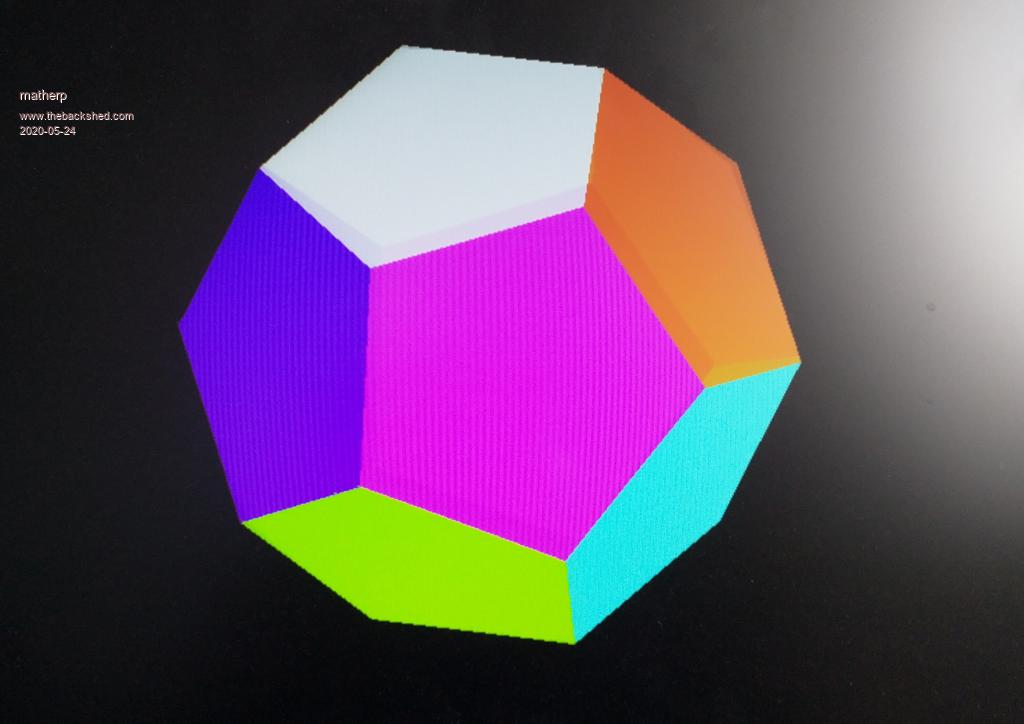option explicit
option default none
page write 1
const edgelength=200 'set the length of the verticies of the dodecahedron
const zlocation=1000 'how far is the center of the dodecahedron away from us
const viewplane=800 'how far is the viewplane away from us
dim float xarr(4),yarr(4)
dim float phi=(1+sqr(5))/2 ' golden ratio
dim float x,y,x1,y1,z1
dim integer col(11), sortorder(11)
dim float q1(4),depth(11),v(4), vout(4)
' data for location of verticies for dodecahedron of edge length 2
data phi,phi,phi
data phi,phi,-phi
data phi,-phi,phi
data -phi,phi,phi
data -phi,-phi,phi
data -phi,phi,-phi
data phi,-phi,-phi
data -phi,-phi,-phi
data 0,-(phi^2),1
data 0,phi^2,1
data 0,-(phi^2),-1
data 0,phi^2,-1
data phi^2,1,0
data -(phi^2),1,0
data phi^2,-1,0
data -(phi^2),-1,0
data 1,0,phi^2
data 1,0,-(phi^2)
data -1,0,phi^2
data -1,0,-(phi^2)
dim float dodec(2,19), ndodec(3,19)
dim integer i,j,k

' read in the coordinates of the verticies and scale
for j=0 to 19
�for i=0 to 2
� �dodec(i,j)=dodec(i,j)*edgelength/2
�next i
next j

'convert coordinates to normalised form
for i=0 to 19
�x1=dodec(0,i): y1=dodec(1,i): z1=dodec(2,i)
�create_vector(x1,y1,z1,v())
�ndodec(0,i)=v(2): ndodec(1,i)=v(3): ndodec(2,i)=v(4): ndodec(3,i)=v(0)
next i

'create a quarternion to rotate 5 degrees about a chosen axis
'play with the x,y,z vector which is the sxis of rotation
create_normalised_quaternion(5,1,0.5,0.25,q1())

'array to hold verticies for each face and its colour
dim integer faces(4,11)
data 10,6,17,19,7,rgb(red)
data 7,19,5,13,15,rgb(blue)
data 6,14,12,1,17,rgb(yellow)
data 19,17,1,11,5,rgb(green)
data 8,2,16,18,4,rgb(magenta)
data 2,14,12,0,16,rgb(cyan)
data 18,16,0,9,3,rgb(brown)
data 4,18,3,13,15,rgb(white)
data 12,0,9,11,1,rgb(gray)
data 13,3,9,11,5,rgb(255,0,128)
data 8,4,15,7,10,rgb(128,0,255)
data 8,2,14,6,10,rgb(128,255,0)
for j=0 to 11
�for i=0 to 4
�next i
next j

do
cls
for i=0 to 19 'rotate coordinates
�v(2)=ndodec(0,i): v(3)=ndodec(1,i): v(4)=ndodec(2,i): v(0)=ndodec(3,i): v(1)=0
�rotate_vector(vout(),v(),q1())
�ndodec(0,i)=vout(2): ndodec(1,i)=vout(3): ndodec(2,i)=vout(4): ndodec(3,i)=vout(0)
next i

' Now see which faces are furthest away by adding up the Z coordinates
for i=0 to 11
�depth(i)=0
�sortorder(i)=i
�for j=0 to 4
� depth(i)=depth(i)+ndodec(2,faces(j,i))
�next j
next i
'
sort depth(),sortorder()

for k=0 to 11
�i=sortorder(11-k) 'get the index to the faces in order of nearest last
�for j=0 to 4
� xarr(j)=ndodec(0,faces(j,i))*viewplane/(ndodec(2,faces(j,i))+zlocation)*ndodec(3,faces(j,i))+MM.HRES/2
� yarr(j)=ndodec(1,faces(j,i))*viewplane/(ndodec(2,faces(j,i))+zlocation)*ndodec(3,faces(j,i))+MM.VRES/2
�next j
�polygon 5,xarr(),yarr(),col(i),col(i)
next k
page copy 1 to 0,b
loop
'
sub create_normalised_quaternion(theta as float,x as float,y as float,z as float,q() as float)
�q(2)=x* sineterm
�q(3)=y* sineterm
�q(4)=z* sineterm
�q(0)=sqr(q!(1)*q(1) + q(2)*q(2) + q(3)*q(3) + q(4)*q(4)) 'calculate the magnitude
�q(1)=q(1)/q(0) 'create a normalised quaternion
�q(2)=q(2)/q(0)
�q(3)=q(3)/q(0)
�q(4)=q(4)/q(0)
�q(0)=1
end sub
'
sub invert_quaternion(n() as float,q() as float)
�n(0)=q(0)
�n(1)=q(1)
�n(2)=-q(2)
�n(3)=-q(3)
�n(4)=-q(4)
end sub
'
sub multiply_quaternion(n() as float,q1() as float,q2() as float)
�local float a1=q1(1),a2=q2(1),b1=q1(2),b2=q2(2),c1=q1(3),c2=q2(3),d1=q1(4),d2=q2(4)
�n(1)=a1*a2-b1*b2-c1*c2-d1*d2
�n(2)=a1*b2+b1*a2+c1*d2-d1*c2
�n(3)=a1*c2-b1*d2+c1*a2+d1*b2
�n(4)=a1*d2+b1*c2-c1*b2+d1*a2
�n(0)=q1(0)*q2(0)
end sub
'
sub create_vector(x as float,y as float ,z as float,v() as float)
�v(0)=sqr(x*x + y*y + z*z)
�v(1)=0
�v(2)=x/v(0)
�v(3)=y/v(0)
�v(4)=z/v(0)
end sub

sub rotate_vector(vnew() as float,v() as float,q() as float)
�local float n(4),iq(4)
�multiply_quaternion(n(),q(),v())
�invert_quaternion(iq(),q())
�multiply_quaternion(vnew(),n(),iq())
end sub

Edited 2020-05-24 00:30 by matherp

GrogsterJoined: 31/12/2012
Location: New Zealand
Posts: 8593
 Posted: 04:22am 24 May 2020Copy link to clipboardPrint this post

Yes, that is very cute!!!!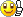Smoke makes things work. When the smoke gets out, it stops!

matherp
GuruJoined: 11/12/2012
Location: United Kingdom
Posts: 7454
 Posted: 12:39pm 24 May 2020Copy link to clipboardPrint this post

Rotating wire-frame bucky ball

Video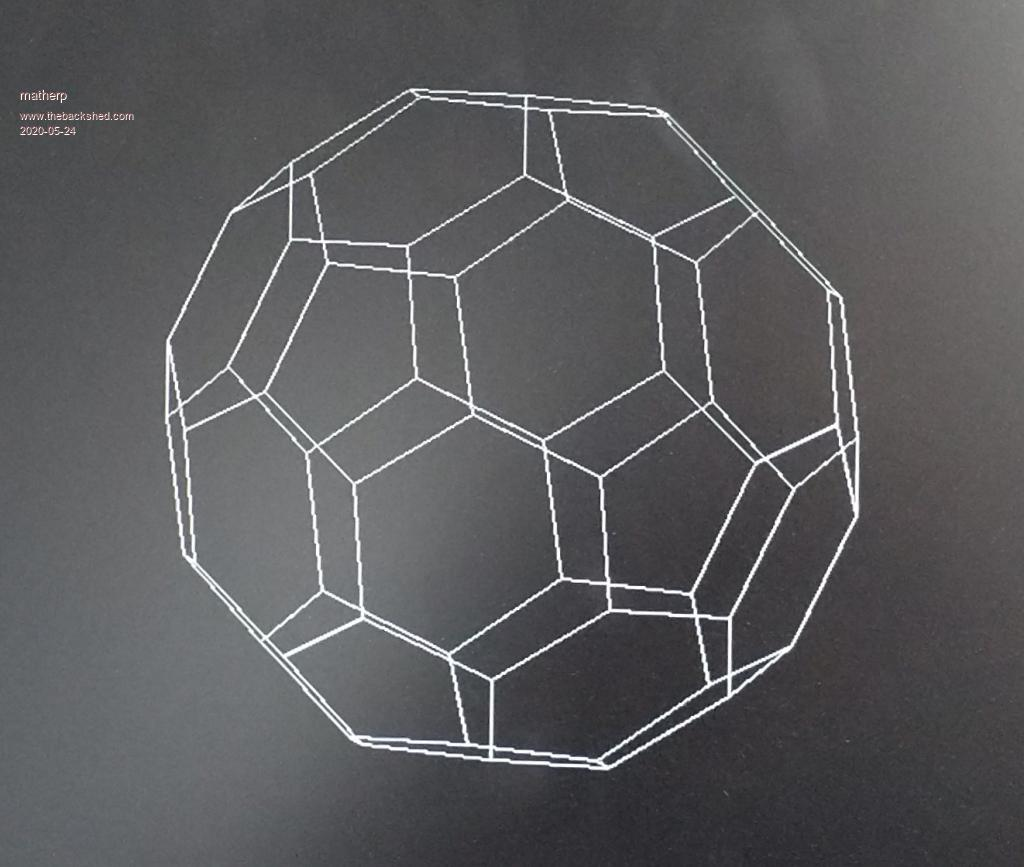option explicit
option default none
page write 1
const edgelength=100 'set the length of the verticies of the ticosahedron
const zlocation=1000 'how far is the center of the ticosahedron away from us
const viewplane=800 'how far is the viewplane away from us
dim float x,y,d
dim float phi=(1+sqr(5))/2
dim float x1,y1,z1
dim integer col(11), sortorder(11)
dim float q1(4),depth(11),v(4), vout(4)
' data for location of verticies for truncated icosahedron of edge length 2
data 0,1,3*phi
data 0,1,-3*phi
data 0,-1,3*phi
data 0,-1,-3*phi
data 1,3*phi,0
data 1,-3*phi,0
data -1,3*phi,0
data -1,-3*phi,0
data 3*phi,0,1
data 3*phi,0,-1
data -3*phi,0,1
data -3*phi,0,-1
data 2,(1+2*phi),phi
data 2,(1+2*phi),-phi
data 2,-(1+2*phi),phi
data 2,-(1+2*phi),-phi
data -2,(1+2*phi),phi
data -2,(1+2*phi),-phi
data -2,-(1+2*phi),phi
data -2,-(1+2*phi),-phi
data (1+2*phi),phi,2
data (1+2*phi),phi,-2
data (1+2*phi),-phi,2
data (1+2*phi),-phi,-2
data -(1+2*phi),phi,2
data -(1+2*phi),phi,-2
data -(1+2*phi),-phi,2
data -(1+2*phi),-phi,-2
data phi,2,(1+2*phi)
data phi,2,-(1+2*phi)
data phi,-2,(1+2*phi)
data phi,-2,-(1+2*phi)
data -phi,2,(1+2*phi)
data -phi,2,-(1+2*phi)
data -phi,-2,(1+2*phi)
data -phi,-2,-(1+2*phi)
data 1,(2+phi),2*phi
data 1,(2+phi),-2*phi
data 1,-(2+phi),2*phi
data 1,-(2+phi),-2*phi
data -1,(2+phi),2*phi
data -1,(2+phi),-2*phi
data -1,-(2+phi),2*phi
data -1,-(2+phi),-2*phi
data (2+phi),2*phi,1
data (2+phi),2*phi,-1
data (2+phi),-2*phi,1
data (2+phi),-2*phi,-1
data -(2+phi),2*phi,1
data -(2+phi),2*phi,-1
data -(2+phi),-2*phi,1
data -(2+phi),-2*phi,-1
data 2*phi,1,(2+phi)
data 2*phi,1,-(2+phi)
data 2*phi,-1,(2+phi)
data 2*phi,-1,-(2+phi)
data -2*phi,1,(2+phi)
data -2*phi,1,-(2+phi)
data -2*phi,-1,(2+phi)
data -2*phi,-1,-(2+phi)

dim float ticos(2,59), nticos(3,59)
dim integer i,j,k
dim integer xs(179),ys(179),xe(179),ye(179)
' read in the coordinates of the verticies and scale
for j=0 to 59
for i=0 to 2
ticos(i,j)=ticos(i,j)*edgelength/2
next i
next j

'Find coordinate pairs that are 100 pixels apart
dim integer linelist(2,59)
for i=0 to 59
k=0
for j=0 to 59
d=sqr((ticos(0,j)-ticos(0,i))^2 + (ticos(1,j)-ticos(1,i))^2 + (ticos(2,j)-ticos(2,i))^2 )
if abs(d-100)<1 then
linelist(k,i)=j
k=k+1
endif
next j
next i

'convert coordinates to normalised form
for i=0 to 59
x1=ticos(0,i): y1=ticos(1,i): z1=ticos(2,i)
create_vector(x1,y1,z1,v())
nticos(0,i)=v(2): nticos(1,i)=v(3): nticos(2,i)=v(4): nticos(3,i)=v(0)
next i

'create a quarternion to rotate 2 degrees about a chosen axis
'play with the x,y,z vector which is the sxis of rotation
create_normalised_quaternion(2,1,0.5,0.25,q1())

do
cls
for i=0 to 59 'rotate coordinates
v(2)=nticos(0,i): v(3)=nticos(1,i): v(4)=nticos(2,i): v(0)=nticos(3,i): v(1)=0
rotate_vector(vout(),v(),q1())
nticos(0,i)=vout(2): nticos(1,i)=vout(3): nticos(2,i)=vout(4): nticos(3,i)=vout(0)
next i

' for every vertex create the lines that radiate from it. This will draw every line twice
j=0
for k=0 to 59
x=nticos(0,k)*viewplane/(nticos(2,k)+zlocation)*nticos(3,k)+MM.HRES/2
y=nticos(1,k)*viewplane/(nticos(2,k)+zlocation)*nticos(3,k)+MM.VRES/2
for i=0 to 2
x1=nticos(0,linelist(i,k))*viewplane/(nticos(2,linelist(i,k))+zlocation)*nticos(3,linelist(i,k))+MM.HRES/2
y1=nticos(1,linelist(i,k))*viewplane/(nticos(2,linelist(i,k))+zlocation)*nticos(3,linelist(i,k))+MM.VRES/2
'store the coordinates for a single line command
xs(j)=x:ys(j)=y:xe(j)=x1:ye(j)=y1:j=j+1
next i
next k
line xs(),ys(),xe(),ye()
page copy 1 to 0,b
loop
'
sub create_normalised_quaternion(theta as float,x as float,y as float,z as float,q() as float)
q(2)=x* sineterm
q(3)=y* sineterm
q(4)=z* sineterm
q(0)=sqr(q!(1)*q(1) + q(2)*q(2) + q(3)*q(3) + q(4)*q(4)) 'calculate the magnitude
q(1)=q(1)/q(0) 'create a normalised quaternion
q(2)=q(2)/q(0)
q(3)=q(3)/q(0)
q(4)=q(4)/q(0)
q(0)=1
end sub
'
sub invert_quaternion(n() as float,q() as float)
n(0)=q(0)
n(1)=q(1)
n(2)=-q(2)
n(3)=-q(3)
n(4)=-q(4)
end sub
'
sub multiply_quaternion(n() as float,q1() as float,q2() as float)
local float a1=q1(1),a2=q2(1),b1=q1(2),b2=q2(2),c1=q1(3),c2=q2(3),d1=q1(4),d2=q2(4)
n(1)=a1*a2-b1*b2-c1*c2-d1*d2
n(2)=a1*b2+b1*a2+c1*d2-d1*c2
n(3)=a1*c2-b1*d2+c1*a2+d1*b2
n(4)=a1*d2+b1*c2-c1*b2+d1*a2
n(0)=q1(0)*q2(0)
end sub
'
sub create_vector(x as float,y as float ,z as float,v() as float)
v(0)=sqr(x*x + y*y + z*z)
v(1)=0
v(2)=x/v(0)
v(3)=y/v(0)
v(4)=z/v(0)
end sub

sub rotate_vector(vnew() as float,v() as float,q() as float)
local float n(4),iq(4)
multiply_quaternion(n(),q(),v())
invert_quaternion(iq(),q())
multiply_quaternion(vnew(),n(),iq())
end sub

matherp
GuruJoined: 11/12/2012
Location: United Kingdom
Posts: 7454
 Posted: 06:16pm 24 May 2020Copy link to clipboardPrint this post

Last one of these more as a proof of the absolute performance of the CMM2 than anything useful - 60 vertices, 32 faces full perspective projection using Painter's algorithm to establish face order. Time for each iteration 85mSec despite a huge amount of maths being done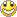Watch the video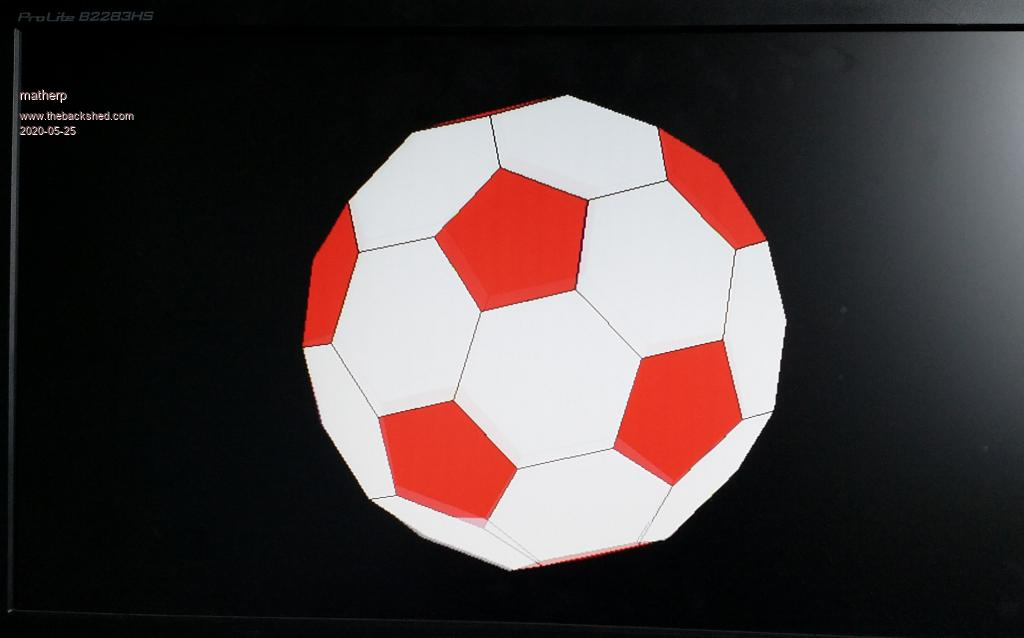option explicit
option default none
mode 2
page write 1
const edgelength=100 'set the length of the verticies of the ticosahedron
const zlocation=1000 'how far is the center of the ticosahedron away from us
const viewplane=800 'how far is the viewplane away from us
dim float x,y,d
dim float phi=(1+sqr(5))/2
dim float x1,y1,z1
dim integer col(11), sortorder(11)
dim float q1(4),depth(11),v(4), vout(4)
' data for location of verticies for ticosahedron of edge length 2
data 0,1,3*phi
data 0,1,-3*phi
data 0,-1,3*phi
data 0,-1,-3*phi
data 1,3*phi,0
data 1,-3*phi,0
data -1,3*phi,0
data -1,-3*phi,0
data 3*phi,0,1
data 3*phi,0,-1
data -3*phi,0,1
data -3*phi,0,-1
data 2,(1+2*phi),phi
data 2,(1+2*phi),-phi
data 2,-(1+2*phi),phi
data 2,-(1+2*phi),-phi
data -2,(1+2*phi),phi
data -2,(1+2*phi),-phi
data -2,-(1+2*phi),phi
data -2,-(1+2*phi),-phi
data (1+2*phi),phi,2
data (1+2*phi),phi,-2
data (1+2*phi),-phi,2
data (1+2*phi),-phi,-2
data -(1+2*phi),phi,2
data -(1+2*phi),phi,-2
data -(1+2*phi),-phi,2
data -(1+2*phi),-phi,-2
data phi,2,(1+2*phi)
data phi,2,-(1+2*phi)
data phi,-2,(1+2*phi)
data phi,-2,-(1+2*phi)
data -phi,2,(1+2*phi)
data -phi,2,-(1+2*phi)
data -phi,-2,(1+2*phi)
data -phi,-2,-(1+2*phi)
data 1,(2+phi),2*phi
data 1,(2+phi),-2*phi
data 1,-(2+phi),2*phi
data 1,-(2+phi),-2*phi
data -1,(2+phi),2*phi
data -1,(2+phi),-2*phi
data -1,-(2+phi),2*phi
data -1,-(2+phi),-2*phi
data (2+phi),2*phi,1
data (2+phi),2*phi,-1
data (2+phi),-2*phi,1
data (2+phi),-2*phi,-1
data -(2+phi),2*phi,1
data -(2+phi),2*phi,-1
data -(2+phi),-2*phi,1
data -(2+phi),-2*phi,-1
data 2*phi,1,(2+phi)
data 2*phi,1,-(2+phi)
data 2*phi,-1,(2+phi)
data 2*phi,-1,-(2+phi)
data -2*phi,1,(2+phi)
data -2*phi,1,-(2+phi)
data -2*phi,-1,(2+phi)
data -2*phi,-1,-(2+phi)
' 12 faces with 5 sides
data 0,28,36,40,32
data 1,29,37,41,33
data 2,30,38,42,34
data 3,31,39,43,35
data 4,12,44,45,13
data 5,14,46,47,15
data 6,16,48,49,17
data 7,18,50,51,19
data 8,20,52,54,22
data 9,21,53,55,23
data 10,24,56,58,26
data 11,27,59,57,25
' 20 faces with 6 sides
data 0,2,34,58,56,32
data 0,2,30,54,52,28
data 1,3,31,55,53,29
data 1,3,35,59,57,33
data 4,6,17,41,37,13
data 4,6,16,40,36,12
data 5,7,19,43,39,15
data 5,7,18,42,38,14
data 8,9,23,47,46,22
data 8,9,21,45,44,20
data 10,11,27,51,50,26
data 10,11,25,49,48,24
data 12,44,20,52,28,36
data 13,45,21,53,29,37
data 14,46,22,54,30,38
data 15,47,23,55,31,39
data 16,48,24,56,32,40
data 17,49,25,57,33,41
data 18,50,26,58,34,42
data 19,51,27,59,35,43
'
dim float zpos(31),zsort(31)
dim float ticos(2,59), nticos(3,59)
dim integer i,j,k,l,m,n
dim integer xs(179),ys(179),xe(179),ye(179)
dim integer index(31),nnum(31)
' read in the coordinates of the verticies and scale
for j=0 to 59
for i=0 to 2
ticos(i,j)=ticos(i,j)*edgelength/2
next i
next j
'
dim integer xarr(179),yarr(179)
dim integer nv(31)=(5,5,5,5,5,5,5,5,5,5,5,5,6,6,6,6,6,6,6,6,6,6,6,6,6,6,6,6,6,6,6,6)
dim integer np(31)=(0,1,2,3,4,5,6,7,8,9,10,11,0,1,2,3,4,5,6,7,8,9,10,11,12,13,14,15,16,17,18,19)
dim integer nd(31)
dim integer f5(4,11), f6(5,19)
dim integer ncol(31)
for j=0 to 11
for i=0 to 4
next i
next j
for j=0 to 19
for i=0 to 5
next i
next j

'convert coordinates to normalised form
for i=0 to 59
x1=ticos(0,i): y1=ticos(1,i): z1=ticos(2,i)
create_vector(x1,y1,z1,v())
nticos(0,i)=v(2): nticos(1,i)=v(3): nticos(2,i)=v(4): nticos(3,i)=v(0)
next i

'create a quarternion to rotate 4 degrees about a chosen axis
'play with the x,y,z vector which is the sxis of rotation
create_normalised_quaternion(4,1,0.5,0.25,q1())

do
cls
for i=0 to 59 'rotate coordinates
v(2)=nticos(0,i): v(3)=nticos(1,i): v(4)=nticos(2,i): v(0)=nticos(3,i): v(1)=0
rotate_vector(vout(),v(),q1())
nticos(0,i)=vout(2): nticos(1,i)=vout(3): nticos(2,i)=vout(4): nticos(3,i)=vout(0)
next i
'
' average the z positions for the five sided faces
for k=0 to 11
zpos(k)=0
for i=0 to 4
zpos(k)=zpos(k)+nticos(2,f5(i,k))
next i
zpos(k)=zpos(k)/5
'    index(k)=k
next k
'average the z positions for the 6 sided faces
for k=12 to 31
zpos(k)=0
for i=0 to 5
zpos(k)=zpos(k)+nticos(2,f6(i,k-12))
next i
zpos(k)=zpos(k)/6
'    index(k)=k
next k
' sort the z positions
sort zpos(),index()
'
j=0:m=0
for l=0 to 31
k=index(l)
m=np(k)
nd(l)=nv(k)
if nv(k)=5 then
ncol(l)=rgb(red)
else
ncol(l)=rgb(white)
endif
for i=0 to nv(k)-1
if nv(k)=5 then
xarr(j)=nticos(0,f5(i,m))*viewplane/(nticos(2,f5(i,m))+zlocation)*nticos(3,f5(i,m))+MM.HRES/2
yarr(j)=nticos(1,f5(i,m))*viewplane/(nticos(2,f5(i,m))+zlocation)*nticos(3,f5(i,m))+MM.VRES/2
else
xarr(j)=nticos(0,f6(i,m))*viewplane/(nticos(2,f6(i,m))+zlocation)*nticos(3,f6(i,m))+MM.HRES/2
yarr(j)=nticos(1,f6(i,m))*viewplane/(nticos(2,f6(i,m))+zlocation)*nticos(3,f6(i,m))+MM.VRES/2
endif
j=j+1
next i
next l
polygon nd(),xarr(),yarr(),rgb(black),ncol()
page copy 1 to 0
loop
'
sub create_normalised_quaternion(theta as float,x as float,y as float,z as float,q() as float)
q(2)=x* sineterm
q(3)=y* sineterm
q(4)=z* sineterm
q(0)=sqr(q!(1)*q(1) + q(2)*q(2) + q(3)*q(3) + q(4)*q(4)) 'calculate the magnitude
q(1)=q(1)/q(0) 'create a normalised quaternion
q(2)=q(2)/q(0)
q(3)=q(3)/q(0)
q(4)=q(4)/q(0)
q(0)=1
end sub
'
sub invert_quaternion(n() as float,q() as float)
n(0)=q(0)
n(1)=q(1)
n(2)=-q(2)
n(3)=-q(3)
n(4)=-q(4)
end sub
'
sub multiply_quaternion(n() as float,q1() as float,q2() as float)
local float a1=q1(1),a2=q2(1),b1=q1(2),b2=q2(2),c1=q1(3),c2=q2(3),d1=q1(4),d2=q2(4)
n(1)=a1*a2-b1*b2-c1*c2-d1*d2
n(2)=a1*b2+b1*a2+c1*d2-d1*c2
n(3)=a1*c2-b1*d2+c1*a2+d1*b2
n(4)=a1*d2+b1*c2-c1*b2+d1*a2
n(0)=q1(0)*q2(0)
end sub
'
sub create_vector(x as float,y as float ,z as float,v() as float)
v(0)=sqr(x*x + y*y + z*z)
v(1)=0
v(2)=x/v(0)
v(3)=y/v(0)
v(4)=z/v(0)
end sub

sub rotate_vector(vnew() as float,v() as float,q() as float)
local float n(4),iq(4)
multiply_quaternion(n(),q(),v())
invert_quaternion(iq(),q())
multiply_quaternion(vnew(),n(),iq())
end sub

CaptainBoing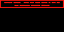GuruJoined: 07/09/2016
Location: United Kingdom
Posts: 1958
 Posted: 08:47pm 24 May 2020Copy link to clipboardPrint this post

just amazing on both your grasp of this and the abilities of the CMM2 you have written Peter.

blows me away to think that the machines this sets out to "copy" were stretched to do this sort of thing in machine code.

An Elite/Frontier in MMBasic would appear to be child's play graphically.

How I wish I had such a machine decades back. I wrote the processor for a weather radar refurb for Plessey with flight data gathering and later examination of weather radar flight data with rotation in a 3D representation versus balloon height (which sadly they weren't interested in). It was a cow to do as it was all FP in 68K assembler. This would be a walk in the park on such a beast.

Paul_L
GuruJoined: 03/03/2016
Location: United States
Posts: 769
 Posted: 01:22am 25 May 2020Copy link to clipboardPrint this post

Andrew ......

was that the 1979 Plessey mini-network system built around a PDP-11/40 installed at the Met Office Radio-Sonde Station, Camborne, Caldwal????

That was an ingenious implementation using the Radar Signal Averaging Unit!!!!

BRAVO!

Paul in NY.

CaptainBoingGuruJoined: 07/09/2016
Location: United Kingdom
Posts: 1958
 Posted: 12:25pm 25 May 2020Copy link to clipboardPrint this post

Hey Paul, you OK?

*** warning thread drift, ignore if you want to

Plessey had a range of weather radars they gave to meteorological authorities around the globe on condition they got the data for the met office in UK so they could do their charts. 4 flights a day, midday/night and six morning/evening. The WF3 was just such a beast, very basic 60's/70's tech (paxolin tag strips with resistors across and tons of wiring).

There was a project (Aladdin) to refit some of them - changes to the head and radome, better electronics and a new microprocessor (Z80) based console replacing the processor which allowed us to modernize the kit and drastically reduce it in size while keeping 100% compatible. Such data was churned out in a standard FM32 PILOT message. Later we added a hardware module for flight data analysis and then PC based - including a very nice version on Amstrad CPC6128s, gutted and embedded in the console... they were a solid, cheap Z80 platform and with memory banking etc. and Floating Point built in the assembler solution was a lot easier to maintain and improve.

I wrote all the software for the meto-processing including sonde flights to churn out FM35 TEMP messages for what became the WF33. Sad case that I am, I still have the code!

It was these flights, saved to disc, that we later added an analysis module (with Commodore Amigas, gutted and embedded) that gave us the really good 3D projection of the flight as wire-frame. Got praise from Plessey and put the skids under VIZ and Visala (competitors) but ultimately was just seen as a toy by the brass. Shame, it was the dawn of visual analysis and modern systems later did it, we were doing it in 1988, but no conviction from the men with the money.

There is a small mention of WF3 (pale blue thing on the end of the bench was the controller we replaced) dunno what processor drove the thing (maybe you can tell from the photo?) and then our WF33 here https://woottonbridgeiow.org.uk/decca-legacy/chapter11.php#11.10
Edited 2020-05-25 22:39 by CaptainBoing

Paul_L
GuruJoined: 03/03/2016
Location: United States
Posts: 769
 Posted: 04:41pm 25 May 2020Copy link to clipboardPrint this post

I'm fine Andrew. My wife is in the hospital with an infection which has nothing to do with COVID-19 which is well south of us. We live well out in the country 75 miles north of NYC. A half mile east there is a modern dairy farm with 250 Holsteins.

Radar equipment generally lasts a long time and there isn't much you can do to improve a magnetron. At Pan Am engineering we had a guy, Bob Ackerly, who was involved in assembling the target radar with linear display which spotted the fleet of aircraft coming to bomb Pearl Harbor but were ignored. Those transceivers on Kauai were still functioning the last time I saw them in 1989! All the improvements come in the signal processing and display areas which was where you were working. I only knew about weather radar through the interfaces we had around the world with local systems.

My specialty was the test equipment code for the airborne autopilot and inertial navigation computers. In the 60s we used a punched tape reader to control a Hawker Siddeley Trace ATE which used a PDP11 to test the Bendix PB20B B707 two axis autopilot. The B747 Bendix autopilot test equipment which showed up in 1968 finally got rid of the punched tape reader. The B747 Delco Carousel INS test equipment was controlled by a program read from an IBM 2314 disk about the size of a washing machine which used the 2316 removable disk pack and stored a little less than 30 MB. These gadgets did not use mainstream computer languages. They used hardware dedicated op codes that controlled the airborne computers directly. It was interesting stuff in its day.

Paul in NY

TassyJimGuruJoined: 07/08/2011
Location: Australia
Posts: 5416
 Posted: 06:24am 30 May 2020Copy link to clipboardPrint this post

Not a demo - more a test but I had to put it somewhere.
This tests the wiring of your 40 way connector.
It needs 28 resistors of the same value. I used 200 ohm but anything from 200 to 2k should do the job.

It first checks to see if the cable is the right way around.
Then we test all digital IN and digital OUT.
The analog in is tested by setting one digital OUT high and one LOW to give a voltage at VCC/2.

My fist go was on a breadboard then I got creative with the soldering iron.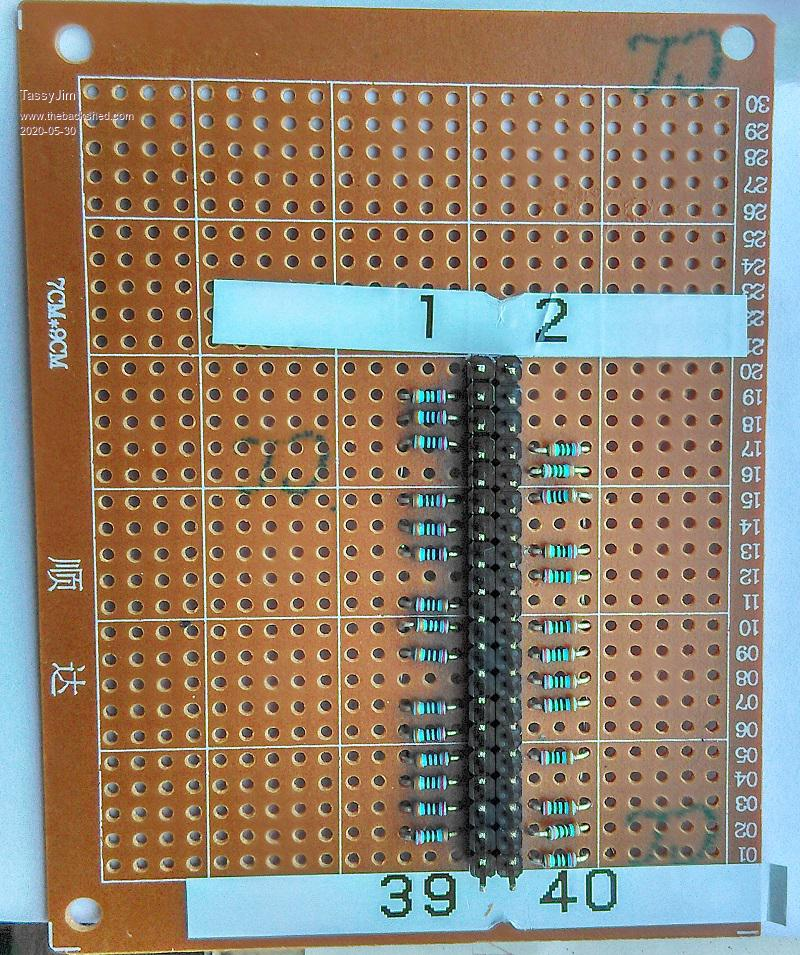' CMM2 pin tester.
' requires a bank of 28 same size resistots (I used 200 ohm)
' connected from each pin in pintest() array to a common point

DIM pintest(27) = (3,5,7,11,13,15,19,21,23,27,29,31,33,35,37,8,10,12,16,18,22,24,26,28,32,36,38,40)
DIM apins(11) = (7,13,15,29,37,8,10,12,16,22,24,26)
DIM INTEGER k, bug
DIM FLOAT v

FOR k = 0 TO 27 ' make sure all pins are floating
SETPIN(pintest(k)), OFF
NEXT k

CLS
' read analog voltage with one pin gnd, all floating then one pin at 3.3V
' header wrong way around will give a narrow range of voltages ~1.16V
PRINT
PRINT "Testing for correct orientation"
SETPIN 13, AIN
SETPIN 3, DOUT
PIN(3) = 0
PRINT "Analog range from ";STR\$(PIN(13),2,2);" > ";
SETPIN 3, OFF
PRINT STR\$(PIN(13),2,2);" > ";
SETPIN 3, DOUT
PIN(3) = 1
v = PIN(13)
PRINT STR\$(v,2,2)
PRINT "A range from 0.2 to 2.9 is good."
PRINT
SETPIN 3, OFF
SETPIN 13, OFF

IF v < 2 THEN
PRINT "It doesn't look right!!"
PRINT "Giving up on the tests."
ELSE
PRINT "Testing Digital IN"
' toggle one pin high/low and check that each pin follows.
SETPIN 40, DOUT
FOR k = 0 TO 26
testpin = pintest(k)
SETPIN testpin , DIN
PIN(40) = 1
IF PIN(testpin ) <> 1 THEN PRINT "Pin ";testpin ;" failed DIN high" : bug = bug+1
PIN(40) = 0
IF PIN(testpin ) <> 0 THEN PRINT "Pin ";testpin ;" failed DIN low" : bug = bug+1
SETPIN testpin , OFF
NEXT k

SETPIN 38, DOUT
testpin = 40
SETPIN testpin , DIN
PIN(38) = 1
IF PIN(testpin ) <> 1 THEN PRINT "Pin ";testpin ;" failed DIN high" : bug = bug+1
PIN(38) = 0
IF PIN(testpin ) <> 0 THEN PRINT "Pin ";testpin ;" failed DIN low" : bug = bug+1
SETPIN testpin , OFF
SETPIN 38, OFF

PRINT
PRINT "Testing Digital OUT"
' toggle each pin in turn and check that output follows.
SETPIN 40, DIN
FOR k = 0 TO 26
testpin = pintest(k)
SETPIN testpin , DOUT
PIN(testpin) = 1
IF PIN(40 ) <> 1 THEN PRINT "Pin ";testpin ;" failed DOUT high" : bug = bug+1
PIN(testpin) = 0
IF PIN(40 ) <> 0 THEN PRINT "Pin ";testpin ;" failed DOUT low" : bug = bug+1
SETPIN testpin , OFF
NEXT k

SETPIN 38, DIN
testpin = 40
SETPIN testpin , DOUT
PIN(testpin) = 1
IF PIN(38 ) <> 1 THEN PRINT "Pin ";testpin ;" failed DOUT high" : bug = bug+1
PIN(testpin) = 0
IF PIN(38 ) <> 0 THEN PRINT "Pin ";testpin ;" failed DOUT low" : bug = bug+1
SETPIN testpin , OFF
SETPIN 38, OFF

PRINT
' set one pin high and one pin low to give VCC/2 at the common point.
SETPIN 3, DOUT
SETPIN 5, DOUT
PIN(3) = 1
PIN(5) = 0

FOR n = 0 TO 11
testpin = apins(n)
SETPIN testpin, AIN
v = PIN(testpin)
IF V < 1.58 OR V > 1.7 THEN PRINT "Pin ";testpin ;" failed AIN - ";v : bug = bug+1
SETPIN testpin, OFF
NEXT n
SETPIN 3, OFF
SETPIN 5, OFF

PRINT
IF bug = 0 THEN
PRINT "All tests completed with no errors"
ELSE
PRINT "A total of ";bug; " errors detected over all tests!!!"
ENDIF
ENDIF
PRINT

This output is with a faulty digital circuit on pin 15.
It failed the digital side but passed the analog due to an internal fault in the CPU.
Testing for correct orientation
Analog range from  0.19 >  1.22 >  2.95
A range from 0.2 to 2.9 is good.

Testing Digital IN
Pin  15 failed DIN low

Testing Digital OUT
Pin  15 failed DOUT high

A total of  2 errors detected over all tests!!!

>

Jim
VK7JH
MMedit   MMBasic Help

lizby
GuruJoined: 17/05/2016
Location: United States
Posts: 2560
 Posted: 11:10am 30 May 2020Copy link to clipboardPrint this post

Are the outer leads of the resistor all ganged? That is what I appear to see through some of the aligned holes.
PicoMite on fruitoftheshed

TassyJimGuruJoined: 07/08/2011
Location: Australia
Posts: 5416
 Posted: 12:23pm 30 May 2020Copy link to clipboardPrint this post

lizby said  Are the outer leads of the resistor all ganged? That is what I appear to see through some of the aligned holes.

Yes
As per the comments in the code
Quote  ' requires a bank of 28 same size resistors (I used 200 ohm)
' connected from each pin in pintest() array to a common point

I should have been clearer.

In the circuit, the numbers in brackets refer to the pin numbers when the cable is arse about.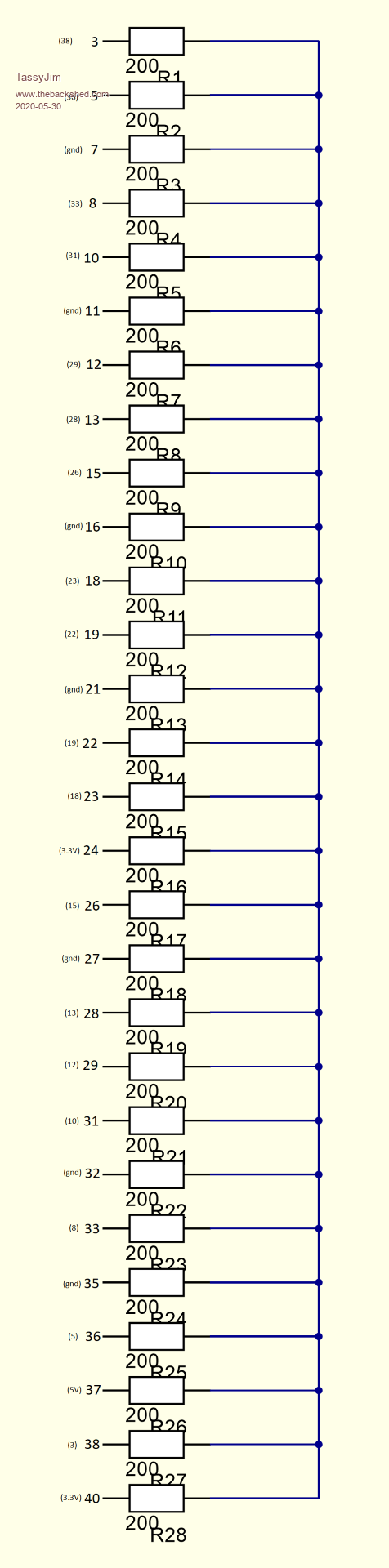Jim
VK7JH
MMedit   MMBasic Help

matherp
GuruJoined: 11/12/2012
Location: United Kingdom
Posts: 7454
 Posted: 01:28pm 30 May 2020Copy link to clipboardPrint this post

Quote  Are the outer leads of the resistor all ganged? That is what I appear to see through some of the aligned holes.

' requires a bank of 28 same size resistots (I used 200 ohm)
' connected from each pin in pintest() array to a common point

Page 1 of 4Print this page